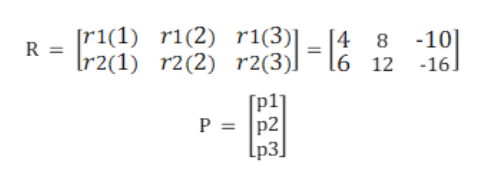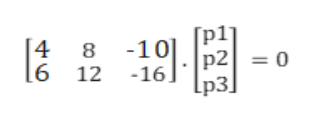An experiment can result in any of the outcomes 1, 2, or 3.  If there are two different wagers with r1(1)=4, r1(2)=8, r1(3)=-10 and r2(1)=6, r2(2)=12, r2(3)=-16, is an arbitrage possible?

Question

An experiment can result in any of the outcomes 1, 2, or 3.  If there are two different wagers with r1(1)=4, r1(2)=8, r1(3)=-10 and r2(1)=6, r2(2)=12, r2(3)=-16, is an arbitrage possible?

Step 1

Let's define two matrix as shown on white board.

The first matrix is R matrix which shows three possible outcomes across two wagers.

The second matrix is the probability matrix, P that has probability of three outcomes as its elements.

Please note that p1, p2 and p3 being probabilities can't be negative and they can't be greater than 1.

Hence, 1 ≥ p1, p2, p3 ≥ 0.help_outlineImage Transcriptionclose[r1(1) r1(2) r1(3)| 8 -10 4 R = Ir2(1) r2(2) r2(3)] 16 12 -16] P = p2 [p3]| fullscreen
Step 2

According to Arbitrage Theorem, an arbitrage exists if we can\'t find a solution to this equation:

R. P = 0

The expanded equation is shown on the white board.help_outlineImage Transcriptionclosep1] 4 8 -10.|p2 0 6 12 -16 [p3] fullscreen
Step 3

So, we get two equations from above:

Equation (1): 4p1 + 8p2 - 10p3 = 0

Equation (2): 6p1 + 12p2 - 16p3 = 0

and we get one ...

Want to see the full answer?

See Solution

Want to see this answer and more?

Our solutions are written by experts, many with advanced degrees, and available 24/7

See Solution
Tagged in